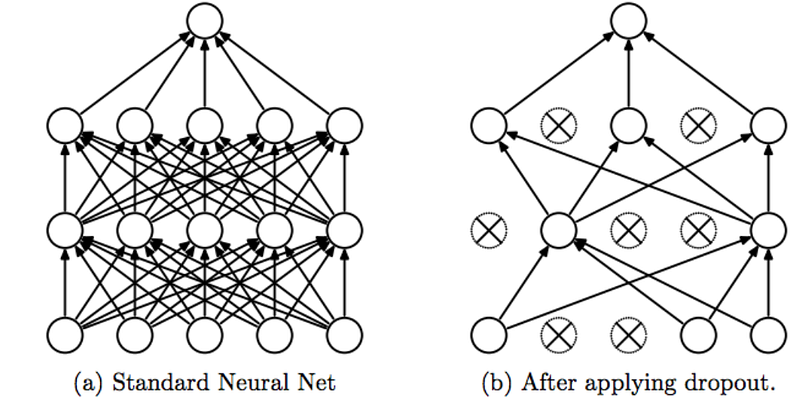# Marko Jerkić

Learner's Deep Learning Blog

## What Is Dropout? – Deep Learning

Overfitting can be a serious problem in deep learning. Dropout is a technique developed to solve this exact problem. It is one of the biggest advancements in deep learning to come out in the last few years.

## What is dropout?

Dropout is a technique for addressing the overfitting problem. The idea is to randomly drop units in a deep neural network.

Learning the relationship between the inputs and the outputs of a dataset is a very complicated procedure. If you have a very small dataset, the relationship maybe a result of noise in the input sample.

Dropout refers to randomly and temporary removing a unit, either in a hidden or a visible layer, and all of its incoming and outgoing connections.

Each node is assigned a probability p, which represents probability of keeping the node. The value is in the range between 0 and 1. For example, you might set for each unit in a hidden layer a keep probability of 0.5. That means that, for each of those units, there is a 50% chance that the unit will stay in the network, and a 50% chance that it will be dropped.Choosing p can be treated as fine tuning; as though p is a hyperparameter.

For nodes in the hidden layers, the optimal value often times seems to be around 0.5. While you could apply the same value for the input layer, the standard practice is to set the keep probability of the input and the output layer to 1.0 or somewhere close to that.

## Dropout and testing

The standard practice is not to use dropout during test time.

If dropout is applied to a unit, than the outgoing weights of that unit are multiplied by p, where p is the keep probability of that unit.## Implementing dropout in Tensorflow

Since Tensorflow is my deep learning library of choice, we’re doing this in Tensorflow:

https://gist.github.com/markojerkic/82e390ba60834335f60c07f01616ea07

Implementing dropout in Tensorflow is as simple as that. There are couple more optional parameters (noise_shape, seed and name). You can check the official documentation for more information on those.

You would apply the dropout function after you apply relu to your logits.